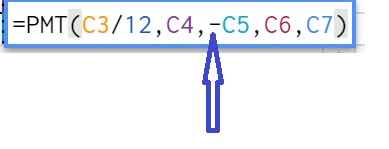## Tuesday, 2 October 2018

The PMT Function is one of the Financial Functions available in The PMT Function in google sheet returns the fixed periodic payment for an investment or loan.

This is an advanced finance function, based on a constant interval of payment like a month, constant interest rate and a constant cash flow. The PMT  function in google sheet is similar to the one available in  MS Excel also.

Practically, accountants and other finance professionals are using the PMT function to calculate monthly payment calculation, creating amortization Schedule and Mortgage Payment schedule etc.

After reading this article, you may able to learn :

• PMT Function in Payment
• PMT Function in Payment to Target
• PMT Function in Creating an Amortization Schedule.
• PMT Function in Creating a Mortgage Schedule.

Syntax

PMT(rate,number_of_periods,present_value,[future_value, end_or_beginning])

Required Parameters
• Rate: The Interest Rate
• Number of Periods: The number of payments to be made
• Present Value: Current Value of Loan or Investment
Optional Parameters
• Future Value: This is the future amount after repaying the loan. If not mentioned, it is considered as zero.
• End or Beginning: This is specifying the payments are due at the beginning (1) or at the end (0) of the period.

When applying the PMT function, we need to use consistent units for Rate and  Number of Periods.

### Example 1-  PMT Function in Payment

Suppose we have a loan amount of \$ 100000 with a loan period of 5 years at 10 % interest.

How can we find the monthly payment?

To ensure the consistent units, we converted the "number of years" to months ie 5 Yrs to 60 months ( 5* 12= 60)

In the formula, the "rate" needs to be divided by 12 to convert to a monthly rate.

Monthly Payment will show as per the table below

It will be a negative figure as the payment is a  cash outward. We can change the result into a positive one by inserting a negative sign (-) before the 'Present Value' in the formula.### Example 2 -  PMT Function in Payment to Target

Suppose we have a loan amount of \$ 100000 and the interest rate is 5 %. The term of the loan is  5 years. The future value will be \$ 10000.The payments are made at the beginning. Then the calculation is as follows

### Example 3 -  PMT Function in Creating an Amortization Schedule.

Amortization is the term used in accounting, means the process of allocation of the cost of an intangible asset over a period of time.

Normally the rate of allocation is giving as an annual rate. If the companies need to charge monthly amortization amount in case they prepare a monthly financial statement.

It also refers to the process of repayment of the loan over a period of time.

How do we prepare the Amortization Schedule by using PMT Function in Google sheet?.

Find the below table of an Amortization Schedule

To prepare an amortization Schedule, We need to use the equation in all the rows and columns as marked 1,2,3,4,5,6,7.

1. Interest Rate: For this row, we need to use the equation to convert the annual rate to a monthly rate. (=C3/12)

2. Term (Monthly): The monthly term is calculated by multiplying the terms in years with 12 months. (=E2*12)

3. Date: This column may have two equation.

• Use the =E3 formula to copy the actual starting date to this cell 'B8'
• In cell 'B9' , apply the equation '=DATE(YEAR(B8),MONTH(B8)+1,DAY(B8))' to automate the date value in the subsequent rows

4. Monthly Payment : this column ,we have to use the PMT Function' =PMT(\$C\$4,\$E\$4,\$C\$2,) ' for total monthly payment .

5. Interest Payment: This column is calculating the interest payment for the month. We need to apply the equation of IPMT Function of google sheet ' =IPMT(\$C\$4,A9,\$E\$4,\$C\$2) '

6. Principal Payment: Principal payment '=C9-D9' is the difference between 'Monthly Payment' and 'Interest Payment'.

7. Loan Remaining: This column may the loan remaining or the balance of intangible asset as per the question. This column has two equations

(a) Colum 'F8', we have to use the formula '=C2' to get the loan Amount from cell C2
(b) Colum 'F9', we have to use the formula '=(F8+E9)' to get the balance amount after the monthly payment.

The '\$' sign along with the cell reference is indicating that the cell was locked. The shortcut is to enter F4 Key after selecting the cell which you wanted to lock.

After entering the above equations, we can see the table as shown below,

Then we need to select the columns from Date to Loan Remaining and drag down to the row numbered as the 60th  month.

Done...that's all, We created an Amortization Schedule or dashboard

Click here to view the file from here.

We can also use this above table for calculating the Mortgage Payments.

Hope you understand how to create PMT Finance Function in Google Sheet.

Please visit the below video also for a clear idea about PMT Finance Function in Google sheet